Supplementary and complementary angles

Chapter 5 Class 7 Lines and Angles
Concept wise

If sum of two angles is 90°, they are complementary

For example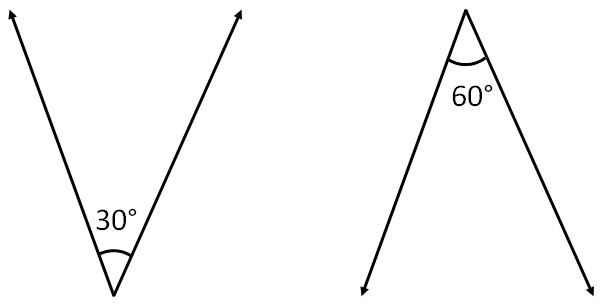30° + 60° = 90°

Since sum of both angles is 90°

So, they are complementary

Are these angles complementary?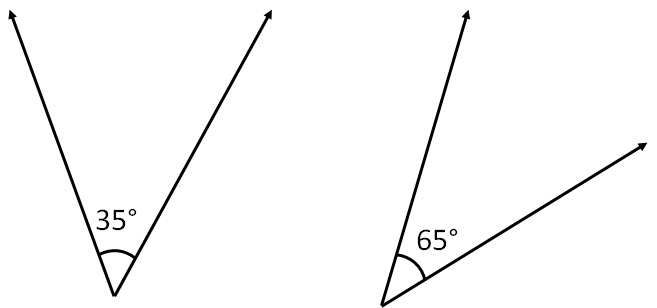35° + 65° = 100°

≠ 90°

Since sum of both angles is not 90°

So, they are not complementary

Are these angles complementary?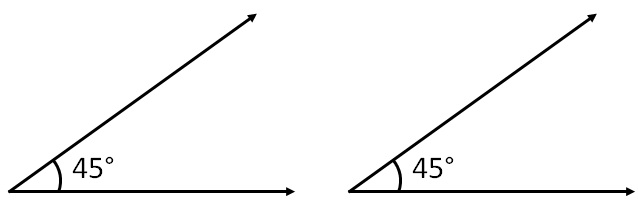45° + 45° = 90°

Since sum of both angle is 90°

So, they are complementary

Are these angles complementary?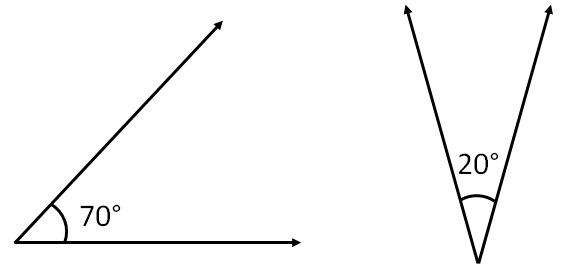70° + 20° = 90°

Since sum of both angle is 90°

So, they are complementary

Are these angles complementary?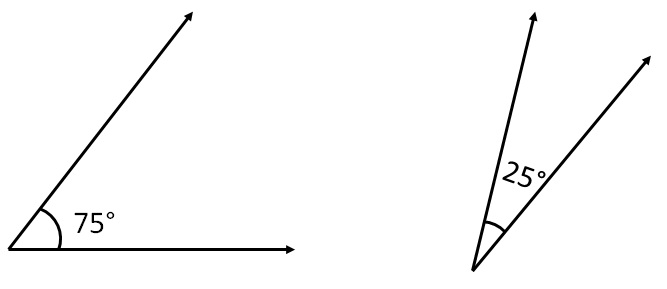75° + 25° = 100°

≠ 90°

Since sum of both angles is not 90°

So, they are not complementary

Are these angles complementary?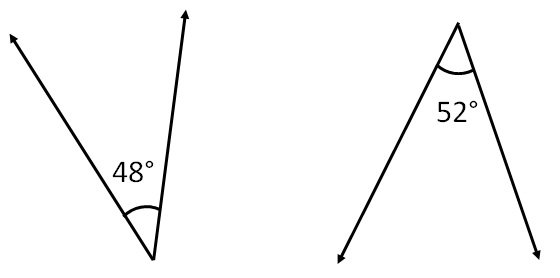48° + 52° = 100°

≠ 90°

Since sum of both angles is not 90°

So, they are not complementary

Are these angles complementary?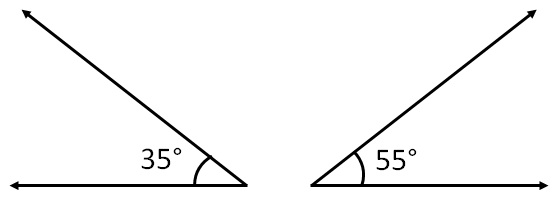35° + 55° = 90°

Since sum of both angles is 90°

So, they are complementary

Learn in your speed, with individual attention - Teachoo Maths 1-on-1 Class